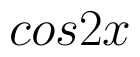Select Page

# CBSE 12 Science Answers for MCQ Maths Differential Equations in English

CBSE 12 Science Answers for MCQ Maths Differential Equations in English to enable students to get Answers in a narrative video format for the specific question.

Expert Teacher provides CBSE 12 Science Answers for MCQ Maths Differential Equations through Video Answers in English language. This video solution will be useful for students to understand how to write an answer in exam in order to score more marks. This teacher uses a narrative style for a question from Differential Equations not only to explain the proper method of answering question, but deriving right answer too.

Please find the question below and view the Answer in a narrative video format.

Question:

## Similar Questions from CBSE, 12th Science, Maths, Differential Equations

Question 1 : Write the sum of the order and degree of the differential equation(View Answer Video)

Question 2 : Find the particular solution of the differential equation :when x = 1,(View Answer Video)

Question 3 :  Find the particular solution of the differential equationgiven thatWhen x =1.  (View Answer Video)

Question 4 : Write the differential equation representing the family of curves y = mx, where m is an arbitrary constant. (View Answer Video)

Question 5 : Solve the differential equationgiven that y = 1 when x = 1. (View Answer Video)

### Integrals

Question 1 : Evaluate :. (View Answer Video)

Question 2 : Write an antiderivative for the function,using the method of inspection. (View Answer Video)

Question 3 : Evaluate :(View Answer Video)

Question 4 : Find :(View Answer Video)

Question 5 : Find :(View Answer Video)

### Application of Integrals

Question 1 : Find the area of the region bounded by the parabolaand y = | x |. (View Answer Video)

Question 2 : Find the area of the smaller region bounded by the ellipseand the straight line 8x + 3y = 12. (View Answer Video)

Question 3 : Find the area of the region. (View Answer Video)

Question 4 : Find the area of the region in the first quadrant enclosed by x-axis, lineand the circle(View Answer Video)

Question 5 : Find the area bounded by the curveand the line x = 4y - 2. (View Answer Video)

### Matrices

Question 1 : Compute:. (View Answer Video)

Question 2 : If, find (x-y). (View Answer Video)

Question 3 :  Find the value of z, if(View Answer Video)

Question 4 : Find the value of z,  from the equation:. (View Answer Video)

Question 5 : Find the value of z,  from the equation:(View Answer Video)